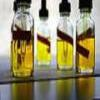#### You may also like### Mathematical Issues for Chemists

A brief outline of the mathematical issues faced by chemistry students.### Reaction Rates

Explore the possibilities for reaction rates versus concentrations with this non-linear differential equation### Catalyse That!

Can you work out how to produce the right amount of chemical in a temperature-dependent reaction?

# What Salt?

##### Age 16 to 18Challenge Level

Having constructed the Born-Haber cycles, and having worked out the relevant data, look at how the two cycles differ. There should be one key point that changed enormously, to make the formation of NaCl$_2$ so unfavourable. Think what the change would be for a NaCl$_3$, and whether this would be offset by the lattice enthalpy. Generally, we can draw a conclusion for NaCl$_n$...

Although the Born-Lande equation will be new to almost everyone, this question involves little more than plugging in given data to the equation. Note that the Born-Lande calculates lattice enthalpies based on a purely electrostatic model, and consequently always underestimates the true lattice enthalpy. What extra factor hasn't been taken into account? Why would this factor increase for larger halides?